# 一、前言

CSS clear both清除产生浮动 ，使用了css float浮动会产生css浮动，这个时候就需要清理清除浮动，就用clear样式属性即可实现。

# 1. clear语法

``clear : none | left|right| both``

# 4. css结构

``````div {
clear: left
}

div {
clear: right
}

div {
clear: both
}``````

# 1. 案例说明

css代码：

``````.div css5 {
width: 500px;
background: #000;
border: 1px solid #F00;
}

.div  css5_left,
.div  css5_right {
border: 1px solid #FFF;
background: #999;
width: 200px;
height: 150px
}
/* css注释：这里为了截图分别，对css代码换行 */

.div  css5_left {
float: left
}
/* css注释：设置浮动靠左 */

.div  css5_right {
float: right
}
/* css注释：设置浮动靠右 */``````

html代码片：

``````<div  class="div css5">
<div  class="div css5_left">float left盒子</div   >
<div  class="div css5_right">float right盒子</div   >
</div>``````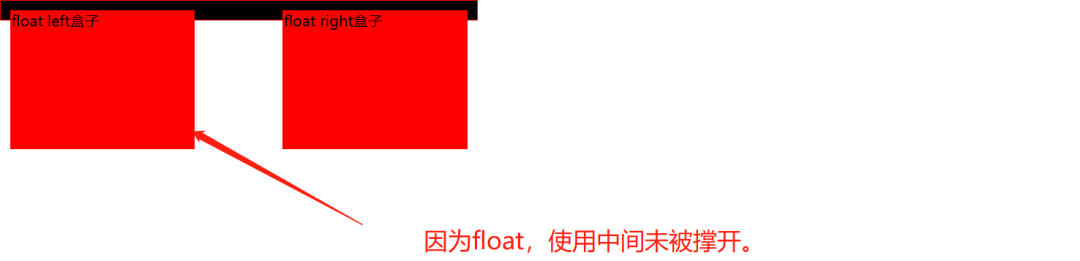# 2. 清除浮动方法

``.clear{ clear:both}``

Html代码中“.div css5”盒子*结束标签前加入代码：

``<div  class="clear"></div>``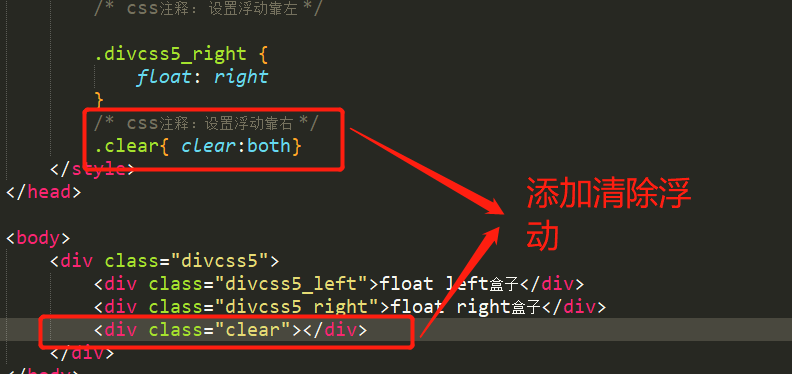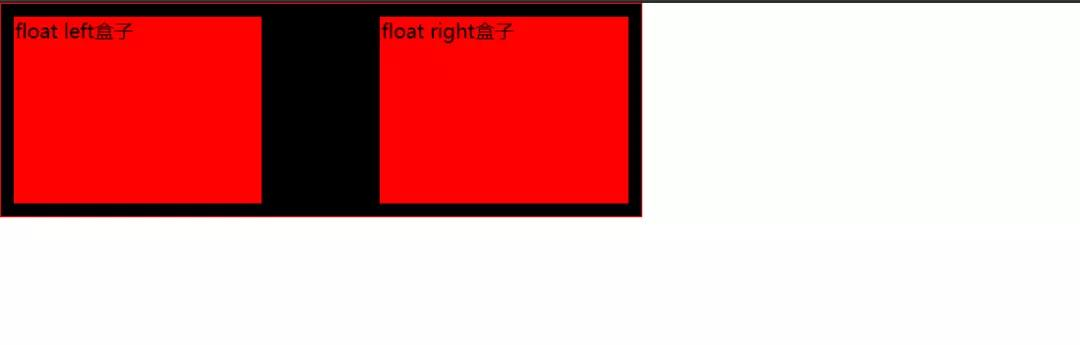# 五、总结

IT共享之家——————- End ——————-

## 手把手教你用Javascript制作随机星星效果图# 1. 创建canvas画布

``````<body>
<canvas id='canvas'></canvas>
</body>``````

# 2. 添加css样式。

``````<style type="text/css">
canvas{
border:2px solid #f00;
}
</style>``````

# 3.1 设置canvas画布大小 ，定义需要变量。

``````<script type="text/javascript">
var _canvas=document.getElementById("canvas")
_canvas.width=500;
_canvas.height=500;
var r,g ,b,a;
</script>``````

# 3.2 产生随机圆。

``````for (var j = 0; j < 150; j++) {
arc.x=Math.floor(Math.random()*_canvas.width);
arc.y=Math.floor(Math.random()*_canvas.height);
arc.r=Math.floor(Math.random()*31+10);
r=Math.ceil(Math.random()*256);
g=Math.ceil(Math.random()*256);
b=Math.ceil(Math.random()*256);
a=Math.random();

darw();
}``````

# 3.3 定义draw()方法，通过画星星公式，将圆形转换成星星状 for 循环产生随机位置星星。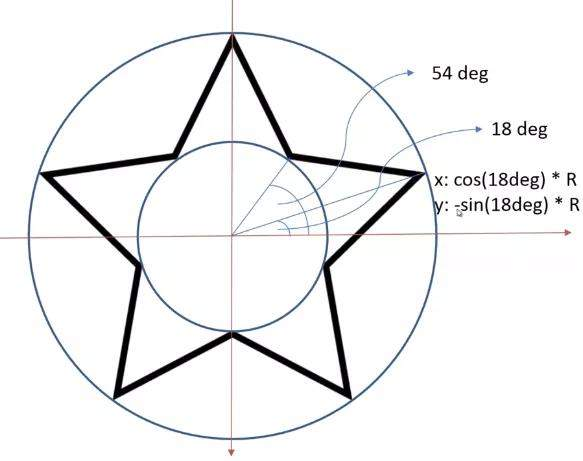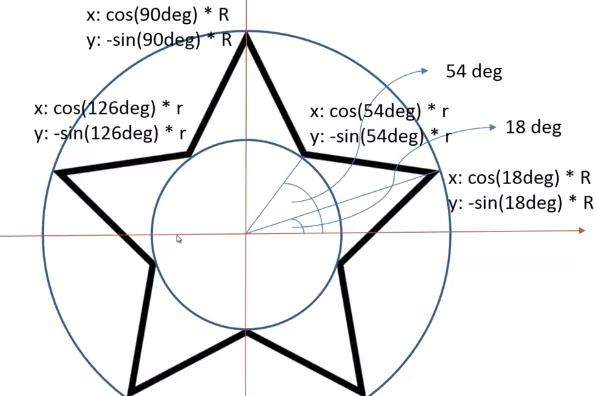``````/* 随机产生星星*/
for (var i = 0; i < 5; i++) {

_ctx.lineTo(Math.cos((18+72*i)/180*Math.PI)*arc.r+arc.x, -Math.sin((18+72*i)/180*Math.PI)*arc.r+arc.y);

_ctx.lineTo(Math.cos((54+72*i)/180*Math.PI)*arc.r/2+arc.x, -Math.sin((54+72*i)/180*Math.PI)*arc.r/2+arc.y);

}``````

# 3.4 随机产生颜色。

Math函数随机产生0-225的RGB值。

``````/* 随机颜色*/
_ctx.fillStyle="rgba(" + r + "," + g + "," + b + "," + a + ")";
_ctx.fill();
_ctx.strokeStyle="rgba(" + r + "," + g + "," + b + "," + a + ")";
_ctx.stroke();
}``````

# 3.5. 调用draw()方法实现功能。

``darw();``

# 五、效果展示

1、点击f12运行到浏览器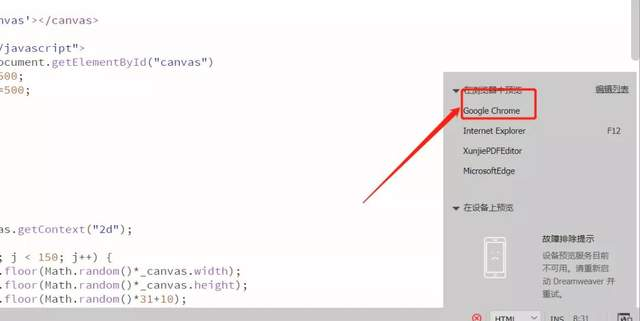2、每次刷新网页，随机产生不一样的星星和随机颜色。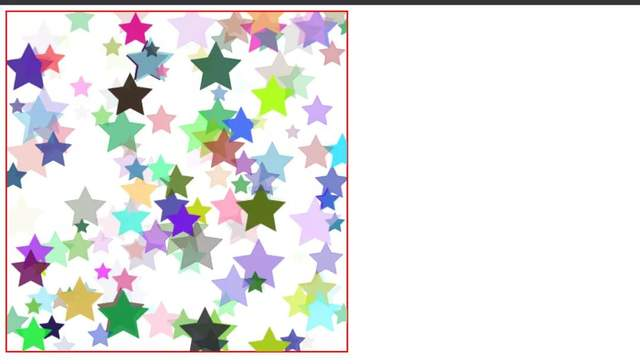# 六、总结

1. 本项目利用canvas画布，实现星星图的效果，以及在运用javascript产生星星效果时，遇到的一些难点进行了分析及提供解决方案。
2. 欢迎大家积极尝试，有时候看到别人实现起来很简单，但是到自己动手实现的时候，总会有各种各样的问题，切勿眼高手低，勤动手，才可以理解的更加深刻。
3. 代码很简单，希望对你有所启发。

IT共享之家——————- End ——————

## 一篇文章教会你用Python爬取淘宝评论数据（写在记事本）

【一、项目简介】

【二、项目准备工作】

1. 准备Pycharm，下载安装等，可以参考这篇文章：Python环境搭建—安利Python小白的Python和Pycharm安装详细教程

2. 爬取商品地址，如下所示：

``https://detail.tmall.com/item.htm?spm=a230r.1.14.1.55a84b1721XG00&id=552918017887&ns=1&abbucket=17``

3. 需要下载几个库，如何下载呢？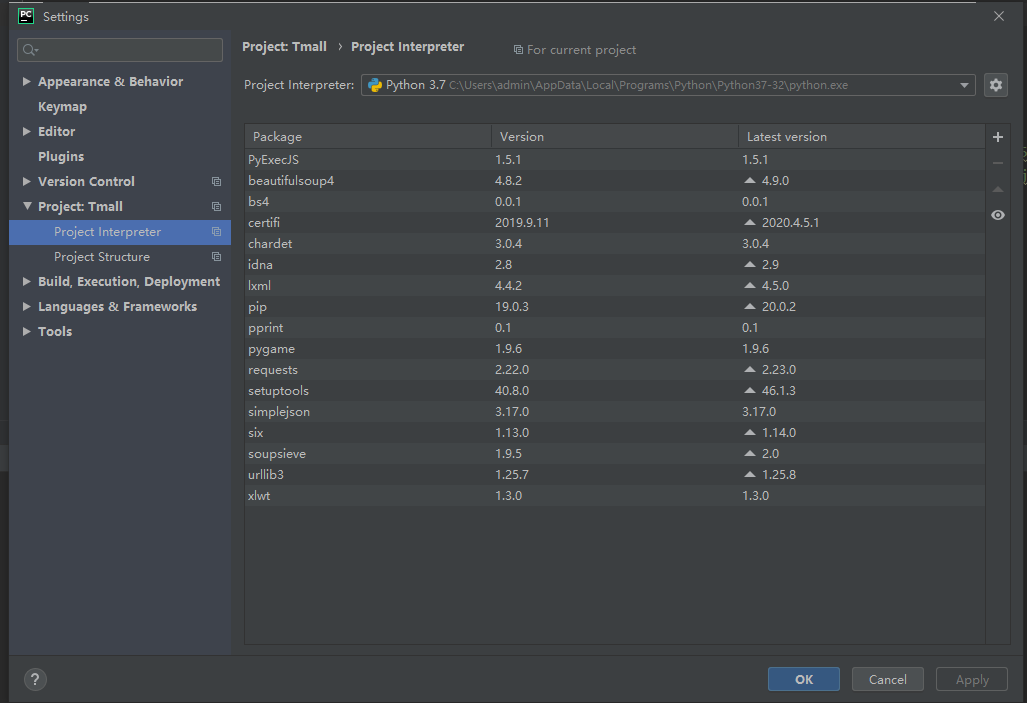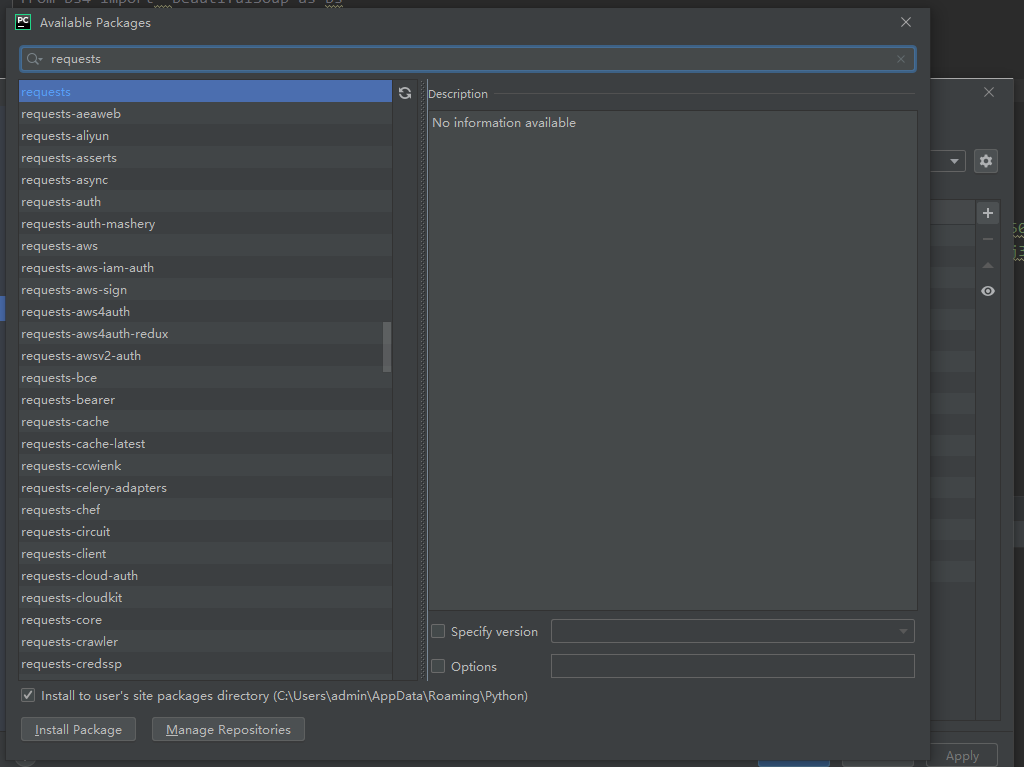【三、项目实现】

1. 导入需要的库

``````import requests
from bs4 import  BeautifulSoup as bs
import json
import csv
import re``````

2. 需要登录淘宝网，选择谷歌浏览器选择开发者工具或按F12有个Network选项，查找list_detail_rate.htm?文件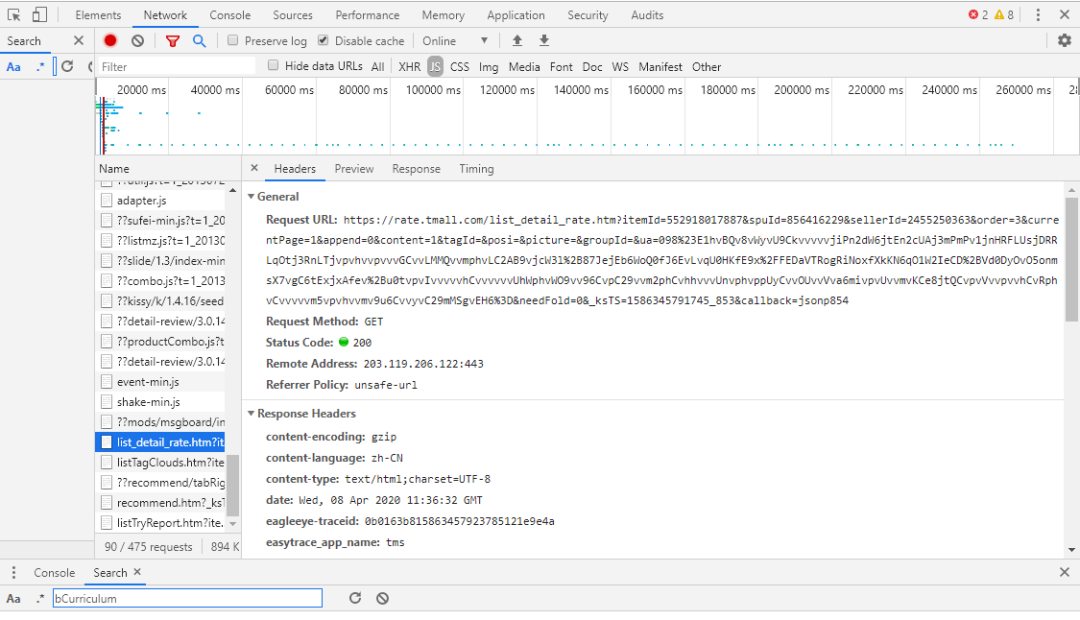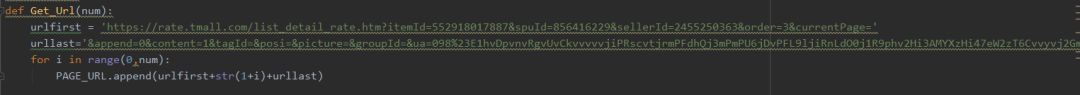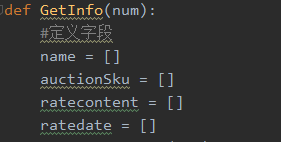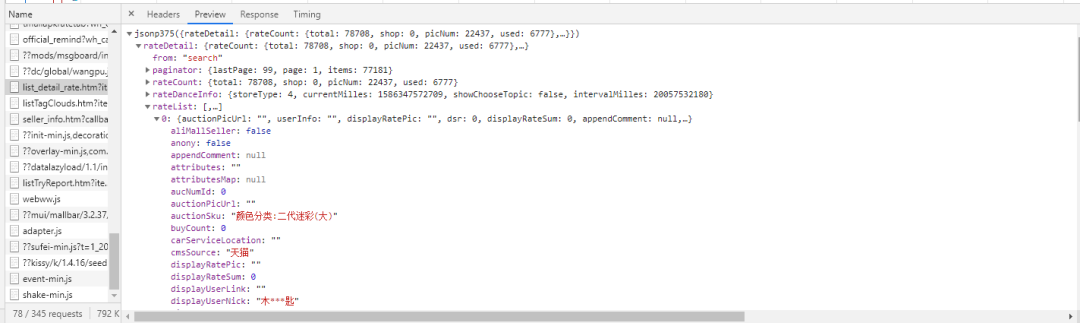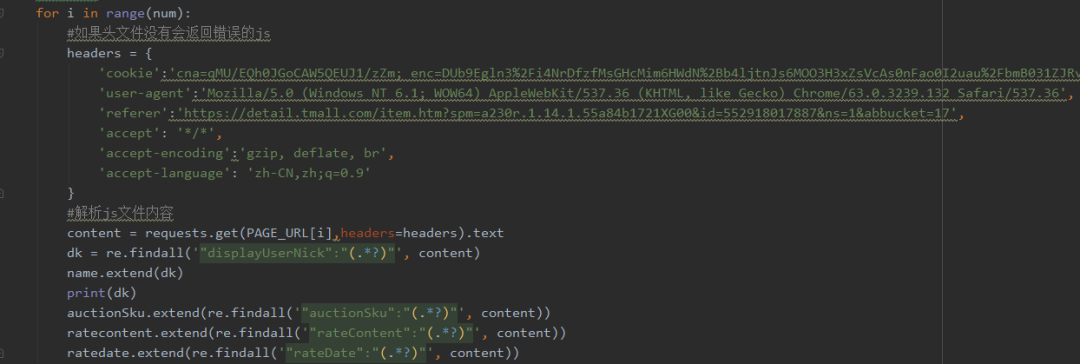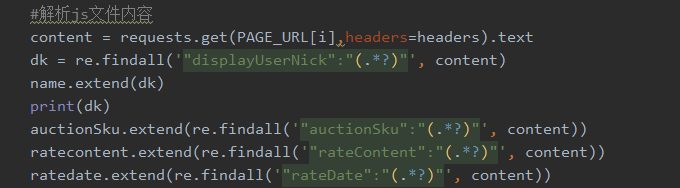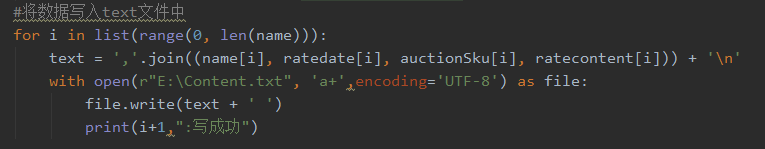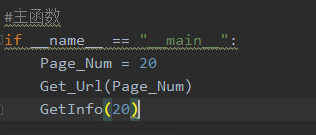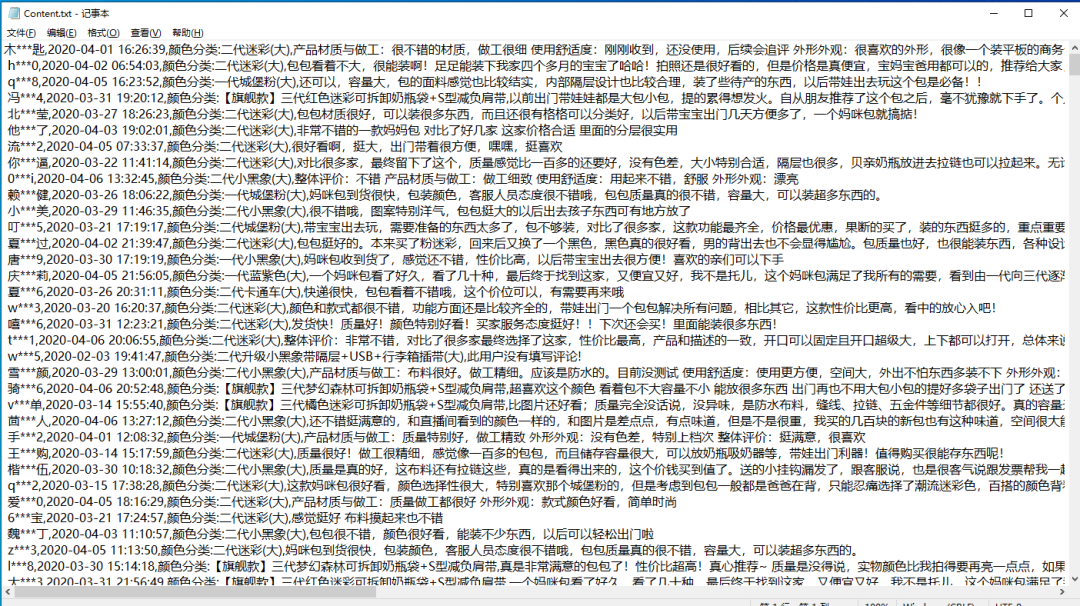【四、总结】

1. 本文基于Python网络爬虫，采集了淘宝商品的评价，方法行之有效。但是建议不要抓取太多，以免对服务器造成压力。

2. 如果需要本文源码，请在公众号后台回复“淘宝评论”获取。

IT共享之家——————- End ——————-